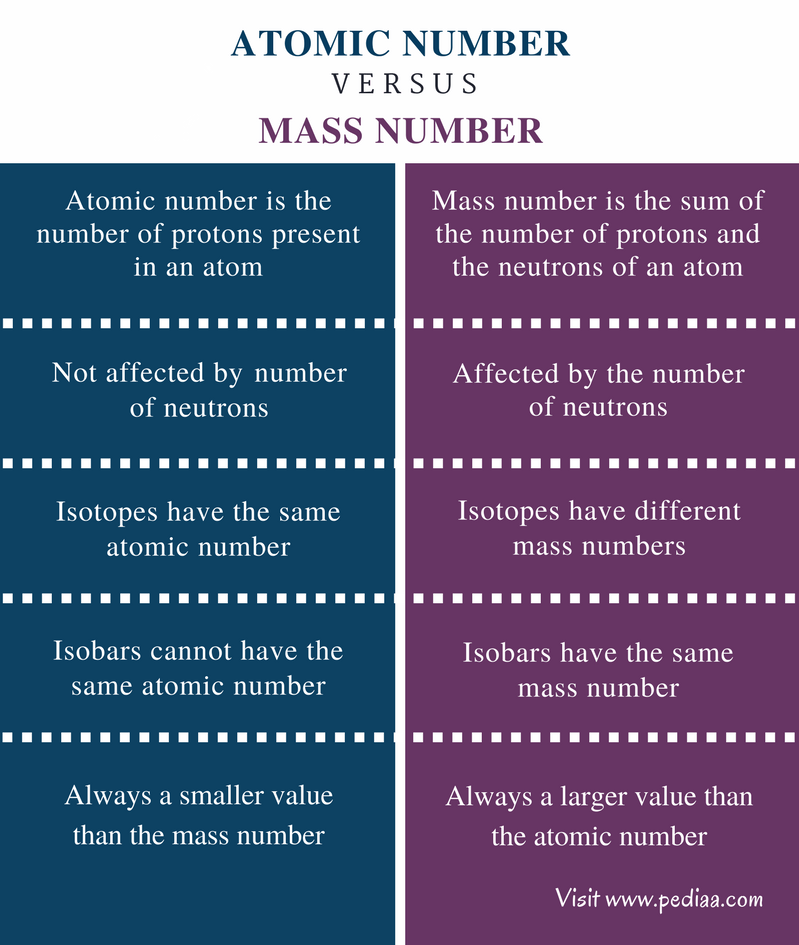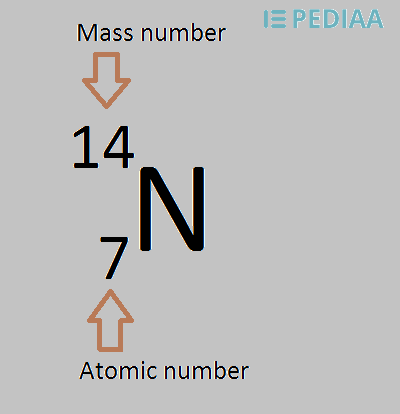# Difference Between Atomic Number and Mass Number

## Main Difference – Atomic Number vs Mass Number

Atoms are composed of electrons, protons, and neutrons. Protons and neutrons together make the nucleus of an atom. When measuring the mass of an atom, we actually measure the mass of the nucleus. That is because the mass of an electron is negligible when compared to a proton or a neutron. The atomic number and the mass number of an atom are two important terms because these terms provide us with details about the number of protons and the number of neutrons present in an atom. The main difference between atomic number and mass number is that the atomic number indicates the number of protons present in an atom whereas, the mass number indicates the sum of the number of protons and the number neutrons present in an atom.

### Key Areas Covered

1. What is Atomic Number

– Definition, Explanation with Examples
2. What is Mass Number

– Definition, Explanation with Examples
3. What is the Difference Between Atomic Number and Mass Number

– Comparison of Key Differences

Key Terms: Atom, Atomic Number, Electron, Isotope, Mass Number, Neutron, Nucleus, Proton## What is Atomic Number

Atomic number is the number of protons present in an atom. The protons are in the nucleus of an atom. The number of protons in an atom is a unique property for a chemical element. Therefore, we can identify a given chemical element by finding the number of protons present in its atom.  Each and every atom has at least one proton. For example, hydrogen atom has only one proton.

Since the atomic number is the number of protons, the atomic number has no units. For example, the number of protons in Helium is 2. Therefore, the atomic number of Helium is 2. Usually, the atomic number is given along with the symbol of the chemical element in a specific way. An example is given below.Figure 1: The atomic number is given in front of the chemical symbol in a lower case number.

Isotopes are atoms that have the same number of protons but different numbers of neutrons. Since these isotopes have the same number of protons, they belong to the same chemical element. Therefore, when we are talking about isotopes, we are talking about the different forms of the same element.

Protons are positively charged subatomic particles. Therefore, the atomic number indicates the total positive charge of a nucleus. In a neutral atom, the number of protons is equal to the number of electrons, because, in order to be neutral, all the positive charges should be neutralized from the negative charges of the electrons. Therefore, the atomic number is equal to the number of electrons present in a neutral atom.

The atomic number of an element can be changed if that element is radioactive. That is because, when some elements undergo radioactivity, protons are converted into different subatomic particles or neutrons may be converted into protons. Either way, it changes the number of protons present in an atom. This changes into a different element (change in atomic number changes the element).

## What is Mass Number

Mass number is the sum of the number of protons and the neutrons of an atom. This means the mass number is the total number of subatomic particles which are present in the nucleus of an atom. A proton or a neutron alone is called a nucleon. Therefore, the mass number can also be given as the total number of nucleons present in the nucleus of an atom.

This is called the mass number because it determines the mass of an atom. The mas of an atom is the total mass of the protons and the neutrons of that atom. That is because the mass of an electron is negligible when compared to a proton or a neutron.

Since the number of protons and the number of neutrons are equal in stable atoms, the mass number of an atom is approximately equal to twice the atomic number. However, in isotopes, the mass number may or may not equal to twice of the atomic number. This is because the isotopes have the same number of protons but a different number of neutrons. Therefore, in those, the mass number is different from that of the expected value.Figure 2: The major isotopes of hydrogen and their mass numbers.

Apart from that, there are some substances called isobars. These are atoms of different elements that have the same mass number, but they are different in their atomic numbers. For example, Chlorine-37 and Argon-37 have the same mass number. Therefore, they are isobars.

As atoms often undergo alpha decay that eliminates two protons and two neutrons from a radioactive nucleus, the mass number of elements can change accordingly. Then the mass number is changed by 4 units.

## Difference Between Atomic Number and Mass Number

### Definition

Atomic Number: Atomic number is the number of protons present in an atom.

Mass Number: Mass number is the sum of the number of protons and the neutrons of an atom.

### Number of Neutrons

Atomic Number: The number of neutrons does not affect the atomic number of an atom.

Mass Number: The number of neutrons does not affect the mass number of an atom.

### Isotopes

Atomic Number: Isotopes have the same atomic number.

Mass Number: Isotopes have different mass numbers.

### Isobars

Atomic Number: Isobars cannot have the same atomic number.

Mass Number: Isobars have the same mass number.

### Value

Atomic Number: The atomic number is always a smaller value than the mass number.

Mass Number: The mass number is always a larger value than the atomic number.

### Conclusion

Atomic number and mass number are fundamental details about an atom. The atomic number is a unique value for a certain chemical element. But there can be elements having the same mass number. They are called isobars. And also, there can be atoms having the same atomic number with different mass numbers yet belonging to the same element. They are called isotopes. The main difference between atomic number and mass number is that the atomic number indicates the number of protons present in an atom whereas, the mass number indicates the sum of the number of protons and the number neutrons present in an atom.

##### References:

1. “ATOMIC NUMBER AND MASS NUMBERS.” NDT Resource center, Available here. Accessed 13 Sept. 2017.
2. “GCSE Bitesize: Atomic number and mass number.” BBC, Available here. Accessed 13 Sept. 2017.
3. “Atomic number.” Wikipedia, Wikimedia Foundation, 5 Sept. 2017, Available here. Accessed 13 Sept. 2017.

##### Image Courtesy:

1. “Blausen 0530 HydrogenIsotopes” By BruceBlaus – Own work (CC BY 3.0) via Commons Wikimedia